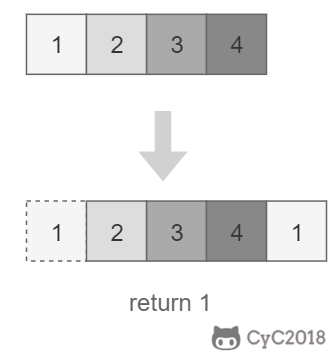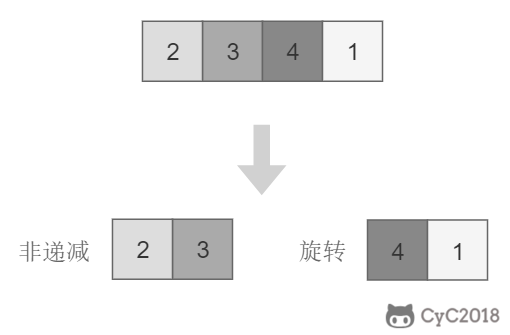# # 11. 旋转数组的最小数字## # 题目描述## # 解题思路• 当 nums[m] <= nums[h] 时，表示 [m, h] 区间内的数组是非递减数组，[l, m] 区间内的数组是旋转数组，此时令 h = m；
• 否则 [m + 1, h] 区间内的数组是旋转数组，令 l = m + 1。
``````public int minNumberInRotateArray(int[] nums) {
if (nums.length == 0)
return 0;
int l = 0, h = nums.length - 1;
while (l < h) {
int m = l + (h - l) / 2;
if (nums[m] <= nums[h])
h = m;
else
l = m + 1;
}
return nums[l];
}
``````

``````public int minNumberInRotateArray(int[] nums) {
if (nums.length == 0)
return 0;
int l = 0, h = nums.length - 1;
while (l < h) {
int m = l + (h - l) / 2;
if (nums[l] == nums[m] && nums[m] == nums[h])
return minNumber(nums, l, h);
else if (nums[m] <= nums[h])
h = m;
else
l = m + 1;
}
return nums[l];
}

private int minNumber(int[] nums, int l, int h) {
for (int i = l; i < h; i++)
if (nums[i] > nums[i + 1])
return nums[i + 1];
return nums[l];
}
``````